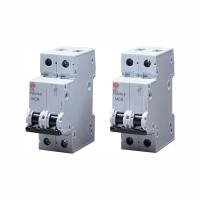6A Double pole MCB B curve 10kA10A Double pole MCB B curve 10kA16A Double pole MCB B curve 10kA20A Double pole MCB B curve 10kA25A Double pole MCB B curve 10kA32A Double pole MCB B curve 10kA40A Double pole MCB B curve 10kA50A Double pole MCB B curve 10kA63A Double pole MCB B curve 10kA6A Double pole MCB C curve 10kA10A Double pole MCB C curve 10kA16A Double pole MCB C curve 10kA20A Double pole MCB C curve 10kA25A Double pole MCB C curve 10kA32A Double pole MCB C curve 10kA40A Double pole MCB C curve 10kA50A Double pole MCB C curve 10kA63A Double pole MCB C curve 10kA6A Double pole MCB D curve 10kA10A Double pole MCB D curve 10kA16A Double pole MCB D curve 10kA20A Double pole MCB D curve 10kA25A Double pole MCB D curve 10kA32A Double pole MCB D curve 10kA40A Double pole MCB D curve 10kA50A Double pole MCB D curve 10kA63A Double pole MCB D curve 10kA

Cat Number Description Pack Unit
B Curve
PSB206-B 6A Double pole MCB B curve 10kA 0 EACHPSB210-B 10A Double pole MCB B curve 10kA 0 EACHPSB216-B 16A Double pole MCB B curve 10kA 0 EACHPSB220-B 20A Double pole MCB B curve 10kA 0 EACHPSB225-B 25A Double pole MCB B curve 10kA 0 EACHPSB232-B 32A Double pole MCB B curve 10kA 0 EACHPSB240-B 40A Double pole MCB B curve 10kA 0 EACHPSB250-B 50A Double pole MCB B curve 10kA 0 EACHPSB263-B 63A Double pole MCB B curve 10kA 0 EACHC Curve
PSB206-C 6A Double pole MCB C curve 10kA 0 EACHPSB210-C 10A Double pole MCB C curve 10kA 0 EACHPSB216-C 16A Double pole MCB C curve 10kA 0 EACHPSB220-C 20A Double pole MCB C curve 10kA 0 EACHPSB225-C 25A Double pole MCB C curve 10kA 0 EACHPSB232-C 32A Double pole MCB C curve 10kA 0 EACHPSB240-C 40A Double pole MCB C curve 10kA 0 EACHPSB250-C 50A Double pole MCB C curve 10kA 0 EACHPSB263-C 63A Double pole MCB C curve 10kA 0 EACHD Curve
PSB206-D 6A Double pole MCB D curve 10kA 0 EACHPSB210-D 10A Double pole MCB D curve 10kA 0 EACHPSB216-D 16A Double pole MCB D curve 10kA 0 EACHPSB220-D 20A Double pole MCB D curve 10kA 0 EACHPSB225-D 25A Double pole MCB D curve 10kA 0 EACHPSB232-D 32A Double pole MCB D curve 10kA 0 EACHPSB240-D 40A Double pole MCB D curve 10kA 0 EACHPSB250-D 50A Double pole MCB D curve 10kA 0 EACHPSB263-D 63A Double pole MCB D curve 10kA 0 EACHCan’t find what your looking for? Then contact us at Electrium or email us.
Wylex Sales
01543 455020
wylex.sales@electrium.co.uk
Wylex Technical
01543 438320
wylex.technical@electrium.co.uk
Export Sales
+44 1543 455049
export@electrium.co.uk